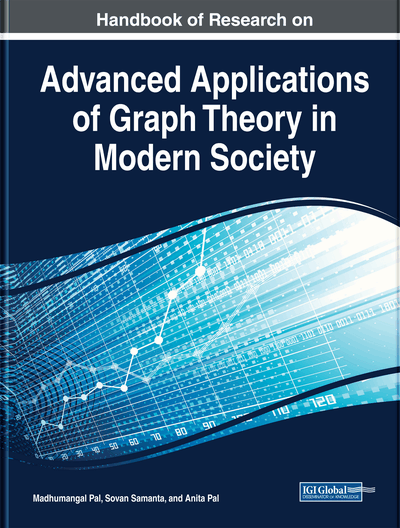# Inverse Sum Indeg Index of Subdivision, t-Subdivision Graphs, and Related Sums

Amitav Doley, Jibonjyoti Buragohain, A. Bharali
DOI: 10.4018/978-1-5225-9380-5.ch005
OnDemand:
(Individual Chapters)
Available
\$37.50
No Current Special Offers

## Abstract

The inverse sum indeg (ISI) index of a graph G is defined as the sum of the weights dG(u)dG(v)/dG(u)+dG(v) of all edges uv in G, where dG(u) is the degree of the vertex u in G. This index is found to be a significant predictor of total surface area of octane isomers. In this chapter, the authors present some lower and upper bounds for ISI index of subdivision graphs, t-subdivision graphs, s-sum and st -sum of graphs in terms of some graph parameters such as order, size, maximum degree, minimum degree, and the first Zagreb index. The extremal graphs are also characterized for their sharpness.
Chapter Preview
Top

## Introduction

A topological index (TI), also known as graph-based molecular descriptor, is a mappingfrom the collection of graphsto the setof real numbers which characterizes numerically a topological structure of a molecular graph numerically. It is a graph invariant which does not depend on the labelling or the pictorial representation of a graph, i.e.,iff the graphs G and H are isomorphic. The values of these indices are very helpful in predicting various physical and chemical properties of a molecular compound, among them boiling point, melting point, strain energy, stability, surface tension of isomers are to name a few. It studies quantitative structure–activity relationship (QSAR) and quantitative structure–property relationship (QSPR) which are used in predicting the biological activities and properties of the chemical compounds.

The first TI was defined by Wiener in the year 1947 (Wiener, 1947), named after his name as Wiener index which is a distance-based TI. Historically Zagreb indices can be considered as the first degree-based topological indices, which came into picture during the study of total π-electron energy of alternant hydrocarbons by Gutman and Trinajstić in 1972 (Gutman & Trinajstić, 1972). Since then many topological indices are proposed and studied based on degree, distance and other parameters of graph. Inverse sum indeg or simply ISI index is relatively a new addition to this wide list of degree based topological indices.

Formally the ISI index can be defined as

.

ISI index was selected by Vukičević and Gašperov (Vukičević & Gašperov, 2010) as a significant predictor of total surface area of octane isomers, and included in the list of 20 (twenty) indices which were selected as significant predictors of physico-chemical properties of a molecular compound out of a list of 148 discrete Adriatic indices considered in their study.

## Complete Chapter List

Search this Book:
Reset